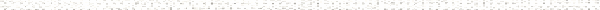A Precalculus Outline

 Chapter 3:  Polynomial FunctionsObjective: Identifying the degree and leading coefficient of a polynomial functionObjective: Identifying the end behavior of graphs of polynomial functionsObjective: Understanding and using the Intermediate Value TheoremObjective: Graphing a polynomial in factored form using end behavior and multiplicities of its zerosObjective: Identifying terms, coefficients, and exponents in a polynomialObjective: Finding domain of a function graphicallyObjective: Finding range of a function graphicallyObjective: Zero of a function> Objective: Finding a relative maximum of a function graphicallyObjective: Finding a relative minimum of a function graphicallyObjective: Finding a local maximum of a function graphicallyObjective: Finding a local minimum of a function graphicallyObjective: Finding the maximum of a function graphicallyObjective: Finding the absolute maximum of a function graphically Section 3.2:  The Factor Theorem and The Remainder TheoremObjective: How to perform synthetic divisionObjective: Using the Remainder TheoremObjective: Using the Factor TheoremObjective: How to perform polynomial long divisionObjective: Using Descartes Rules of Signs to determine the possible number of positive and negative real zeros of a polynomial functionObjective: Using the Rational Zeros Theorem and Synthetic Division to find real zeros of a polynomial functionObjective: Solving polynomial inequalitiesObjective: Synthetic divisionObjective: Factor TheoremObjective: Remainder TheoremObjective: ZeroObjective: MultiplicityObjective: Cost functionObjective: Demand functionObjective: Price-demand functionObjective: Revenue functionObjective: Profit functionObjective: Finding all complex zeros of a polynomial functionObjective: Using the conjugate pairs theorem to find the zeros of a polynomial functionObjective: Understanding the Fundamental Theorem of AlgebraObjective: Forming a polynomial given its zerosObjective: Review of Complex NumbersObjective: The powers of iObjective: Complex Conjugates